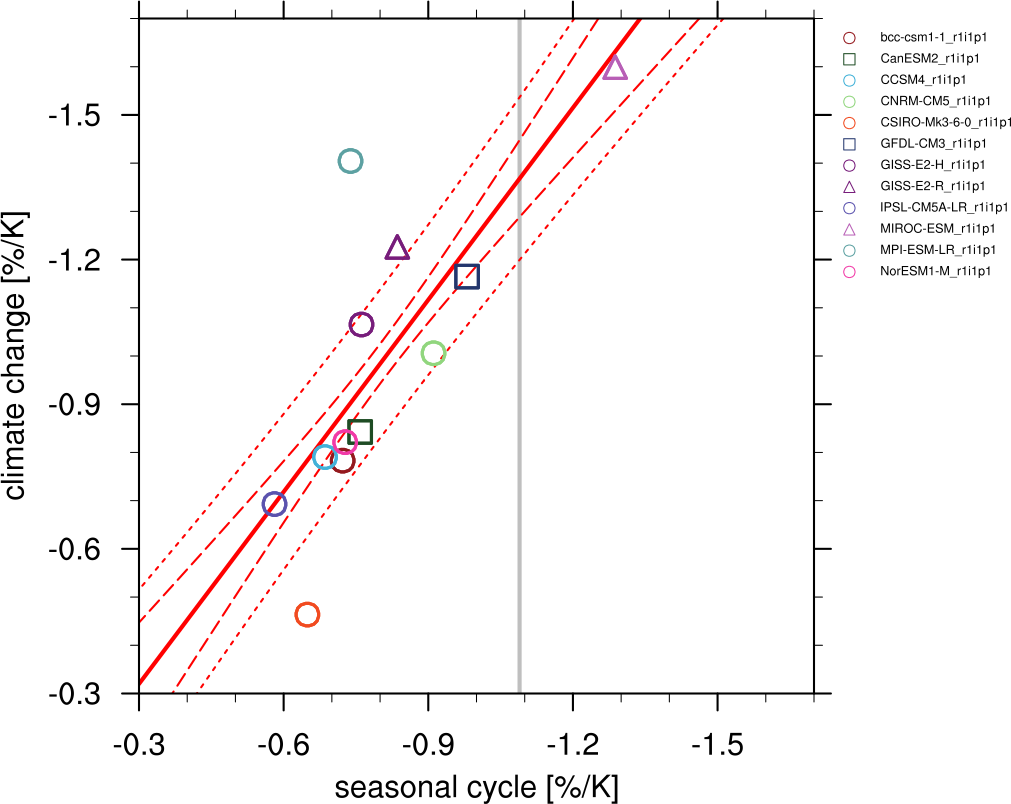# Emergent constraint on snow-albedo effect#

## Overview#

The recipe recipe_snowalbedo.yml computes the springtime snow-albedo feedback values in climate change versus springtime values in the seasonal cycle in transient climate change experiments following Hall and Qu (2006). The strength of the snow-albedo effect is quantified by the variation in net incoming shortwave radiation (Q) with surface air temperature (Ts) due to changes in surface albedo $$\alpha_s$$:

$\left( \frac{\partial Q}{\partial T_s} \right) = -I_t \cdot \frac{\partial \alpha_p}{\partial \alpha_s} \cdot \frac{\Delta \alpha_s}{\Delta T_s}$

The diagnostic produces scatterplots of simulated springtime $$\Delta \alpha_s$$/$$\Delta T_s$$ values in climate change (ordinate) vs. simulated springtime $$\Delta \alpha_s$$/$$\Delta T_s$$ values in the seasonal cycle (abscissa).

Ordinate values: the change in April $$\alpha_s$$ (future projection - historical) averaged over NH land masses poleward of 30°N is divided by the change in April Ts (future projection - historical) averaged over the same region. The change in $$\alpha_s$$ (or Ts) is defined as the difference between 22nd-century-mean $$\alpha_s$$: (Ts) and 20th-century-mean $$\alpha_s$$. Values of $$\alpha_s$$ are weighted by April incoming insolation (It) prior to averaging.

Abscissa values: the seasonal cycle $$\Delta \alpha_s$$/$$\Delta T_s$$ values, based on 20th century climatological means, are calculated by dividing the difference between April and May $$\alpha_s$$: averaged over NH continents poleward of 30°N by the difference between April and May Ts averaged over the same area. Values of $$\alpha_s$$: are weighted by April incoming insolation prior to averaging.

## Available recipes and diagnostics#

Recipes are stored in recipes/

• recipe_snowalbedo.yml

Diagnostics are stored in diag_scripts/emergent_constraints/

• snowalbedo.ncl: springtime snow-albedo feedback values vs. seasonal cycle

## User settings in recipe#

1. Script snowalbedo.ncl

Required settings for script

• exp_presentday: name of present-day experiment (e.g. “historical”)

• exp_future: name of climate change experiment (e.g. “rcp45”)

Optional settings for script

• diagminmax: observational uncertainty (min and max)

• legend_outside: create extra file with legend (true, false)

• styleset: e.g. “CMIP5” (if not set, this diagnostic will create its own color table and symbols for plotting)

• suffix: string to be added to output filenames

• xmax: upper limit of x-axis (default = automatic)

• xmin: lower limit of x-axis (default = automatic)

• ymax: upper limit of y-axis (default = automatic)

• ymin: lower limit of y-axis (default = automatic)

Required settings for variables

• ref_model: name of reference data set

Optional settings for variables

none

Required settings (scripts)

none

Optional settings (scripts)

## Variables#

• tas (atmos, monthly mean, longitude latitude time)

• rsdt (atmos, monthly mean, longitude latitude time)

• rsuscs, rsdscs (atmos, monthly mean, longitude latitude time)

## Observations and reformat scripts#

• ERA-Interim (tas - esmvaltool/cmorizers/data/formatters/datasets/era_interim.py)

• ISCCP-FH (rsuscs, rsdscs, rsdt - esmvaltool/cmorizers/data/formatters/datasets/isccp_fh.ncl)

## Example plots#Fig. 119 Scatterplot of springtime snow-albedo effect values in climate change vs. springtime $$\Delta \alpha_s$$/$$\Delta T_s$$ values in the seasonal cycle in transient climate change experiments (CMIP5 historical experiments: 1901-2000, RCP4.5 experiments: 2101-2200). Similar to IPCC AR5 Chapter 9 (Flato et al., 2013), Figure 9.45a.#